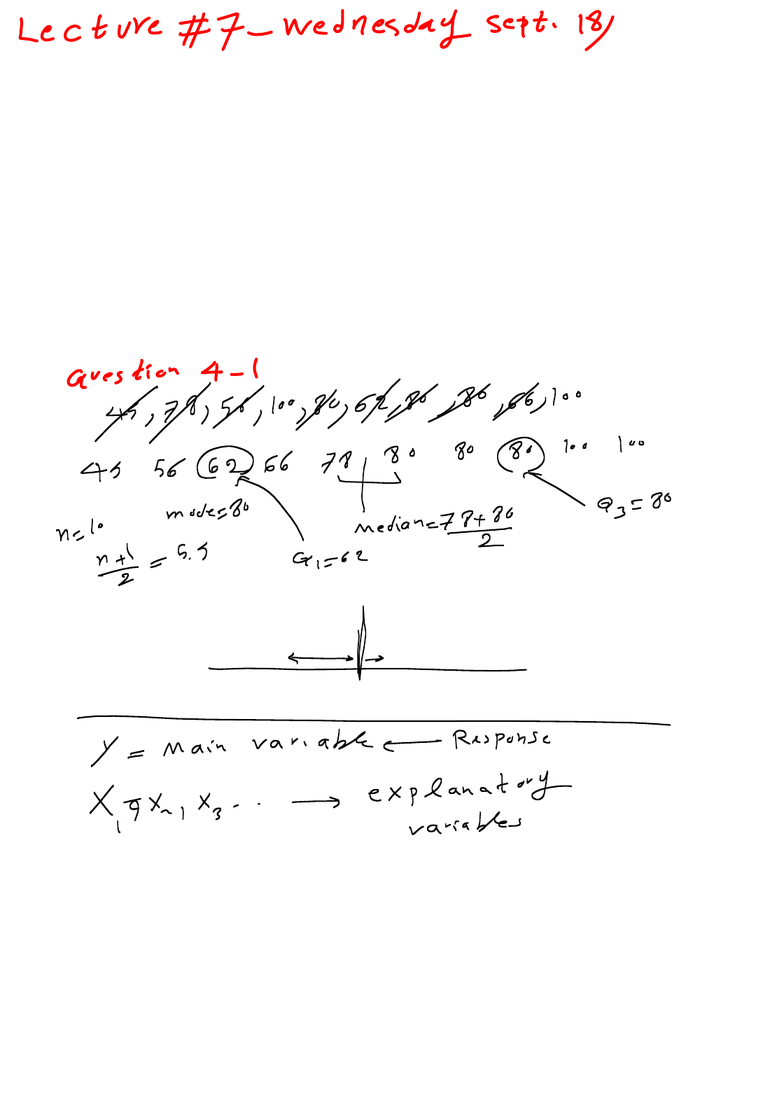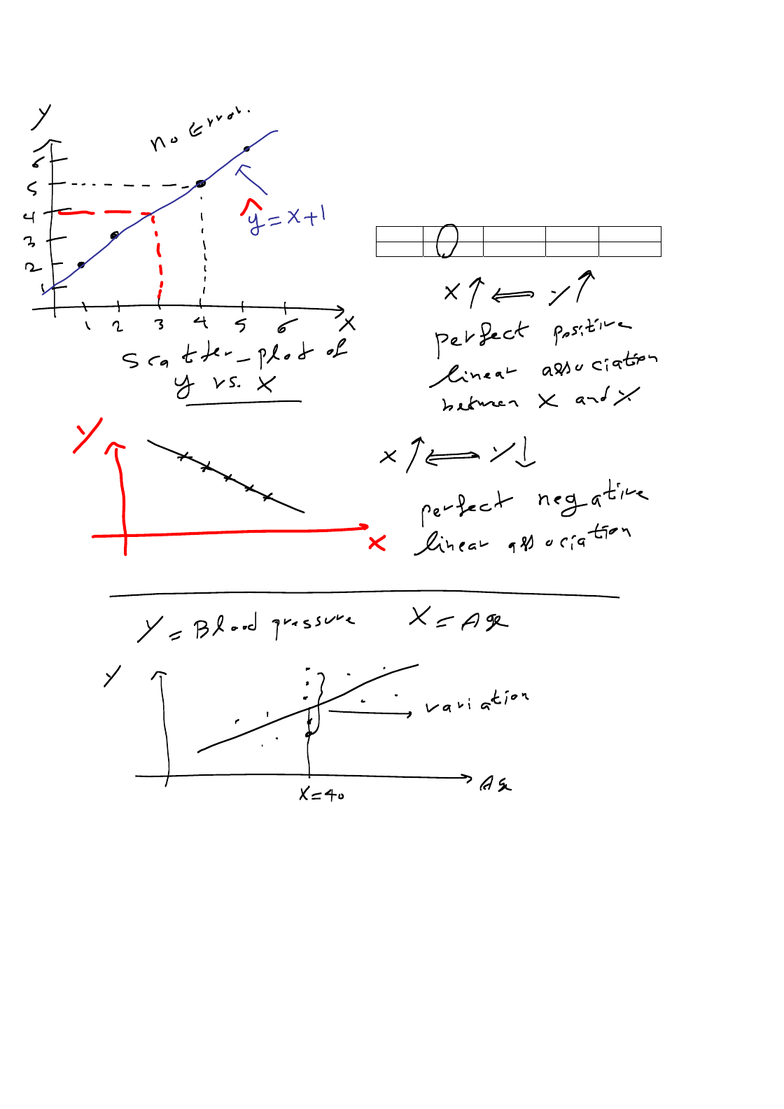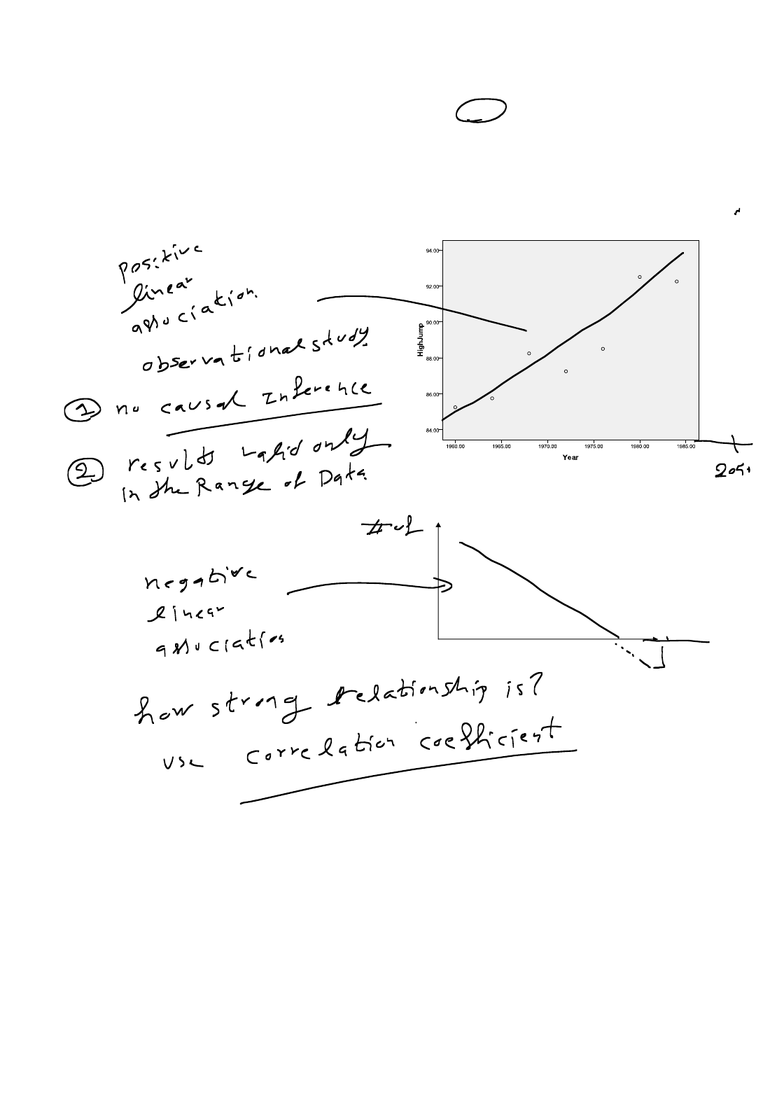Textbook Notes (270,000)
CA (160,000)
U of A (2,000)
BIOCH (20)
Chapter 7-10

# Chapters 7-10.pdf

Department
Biochemistry
Course Code
BIOCH499A
Professor
Gail Amort- Larson
Chapter
7-10

This preview shows pages 1-3. to view the full 29 pages of the document.Chapters 7 – 10: Exploring Relationship Between Variables -
Linear Regression and Correlation
In these chapters, we will quickly talk about
Scatter-plots
Association between two quantitative variables
The purposes of regression and correlation analysis,
How to compute and to interpret a correlation coefficient,
How to compute and to interpret regression equations,
How to predict the outcome of a variable, extrapolating,
Residuals, outliers, influential points,

Only pages 1-3 are available for preview. Some parts have been intentionally blurred.Definition: A scatter-plot is a graphical display for two quantitative
variables. It uses the horizontal axis for the explanatory variable X and the
vertical axis for the response Y. The values of X and Y for a subject are
represented by a point relative to the two axes. The observations for the
n
subjects are
n
points on the scatter-plot.
Example 1: Find the scatter-plot of the following data
x 4 2 5 1
y 5 3 6 2

Only pages 1-3 are available for preview. Some parts have been intentionally blurred.Definition: Two quantitative variables are said to have a positive
association when high value of X tend to occur with high value of Y, and
when low values of X tend to occur with low values of Y. They are said to
have a negative association when high values of one variable tend to pair
with low values of the other variable, and low values of one pair with high
values of the other.
Title: scatter-plot of Olympic gold medal
performances in the men’s high jump from 1960 to
1984.
Sales
Price